# ggwordcloud: a word cloud geom for ggplot2

#### 2023-10-26

`ggwordcloud` provides a word cloud text geom for `ggplot2`. The placement algorithm implemented in C++ is an hybrid between the one of `wordcloud` and the one of `wordcloud2.js`. The cloud can grow according to a shape and stay within a mask. The size aesthetic is used either to control the font size or the printed area of the words. `ggwordcloud` also supports arbitrary text rotation. The faceting scheme of `ggplot2` can also be used. Two functions meant to be the equivalent of `wordcloud` and `wordcloud2` are proposed. Last but not least you can use `gridtext` markdown/html syntax in the labels.

This vignette is meant as a quick tour of its features.

# Package installation

The package can be installed from CRAN by

``install.packages("ggwordcloud")``

or the development version from the github repository

``devtools::install_github("lepennec/ggwordcloud")``

Please check the latest development version before submitting an issue.

# The love / thank you words dataset

Along this vignette, we will use a lovely dataset: a collection of the word love in several language combined with the number of native speakers of those language as well as the total number of speakers. The data have been extracted from wikipedia and is exposed in two data frame of 4 columns: - `lang`: the ISO 649 language code - `words`: the word love in those languages - `native_speakers`: the number of native speakers (in millions) of those languages - `speaker`: the corresponding total number of speakers (in millions) Another dataset with thank you in several languages is also available. The first one `love_words` (`thankyou_words`) contains 147 (133) different languages while the second `love_words_small` (`thankyou_words_small`) contains the 34 (34) languages having more than 50 millions speakers.

``````library(ggwordcloud)
#> Le chargement a nécessité le package : ggplot2
data("love_words_small")
data("love_words")``````

# Word cloud

The `geom_text_wordcloud` geom constructs a word cloud from a list of words given by the `label` aesthetic:

``````set.seed(42)
ggplot(love_words_small, aes(label = word)) +
geom_text_wordcloud() +
theme_minimal()``````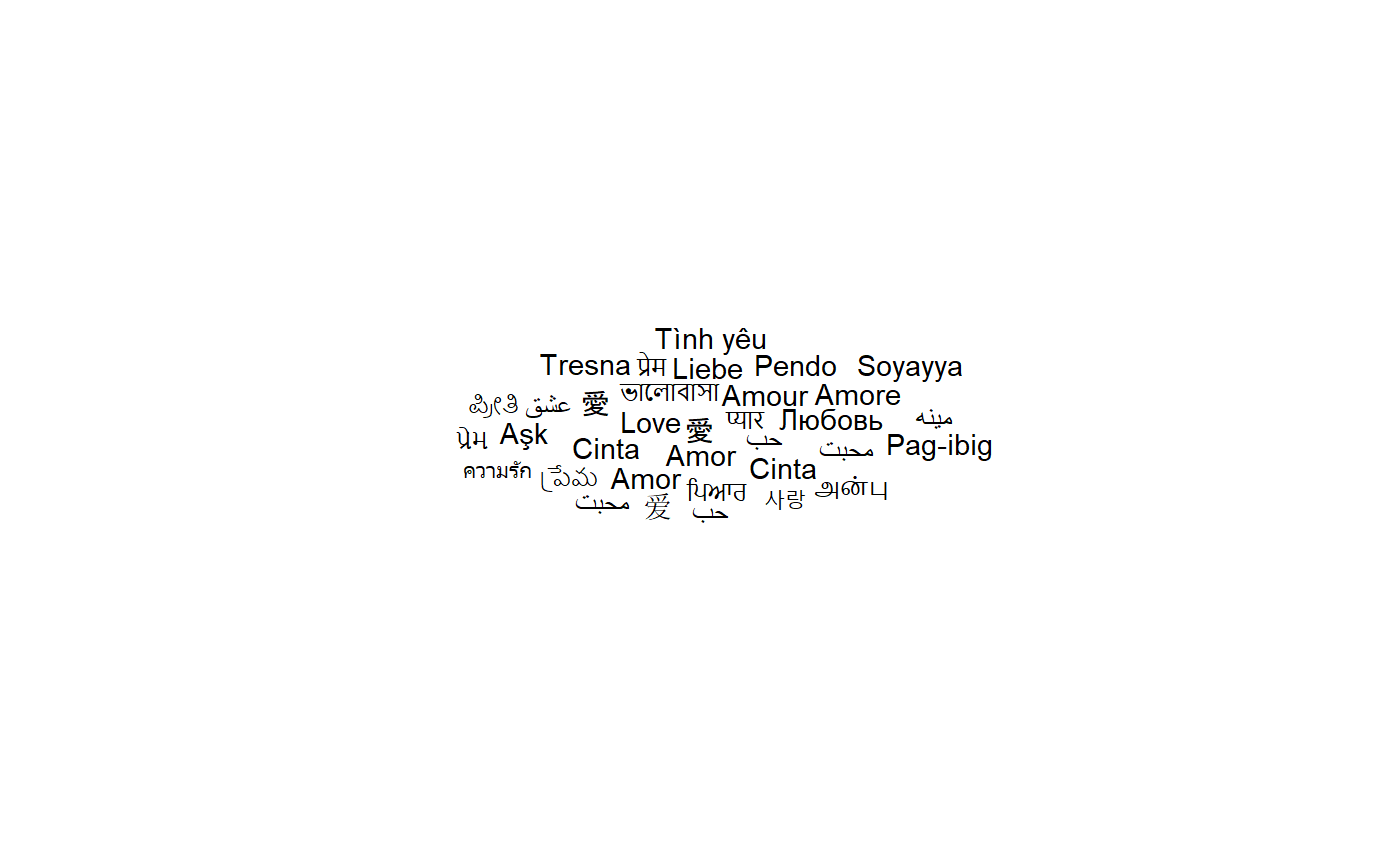Note that we have used `theme_minimal()` to display the words and nothing else. The word cloud is, by default, centered and the words are placed along a spiral in a way they do not overlap.

Because there is some randomness in the placement algorithm, the same command can yield a different result when using a different random seed:

``````set.seed(43)
ggplot(love_words_small, aes(label = word)) +
geom_text_wordcloud() +
theme_minimal()``````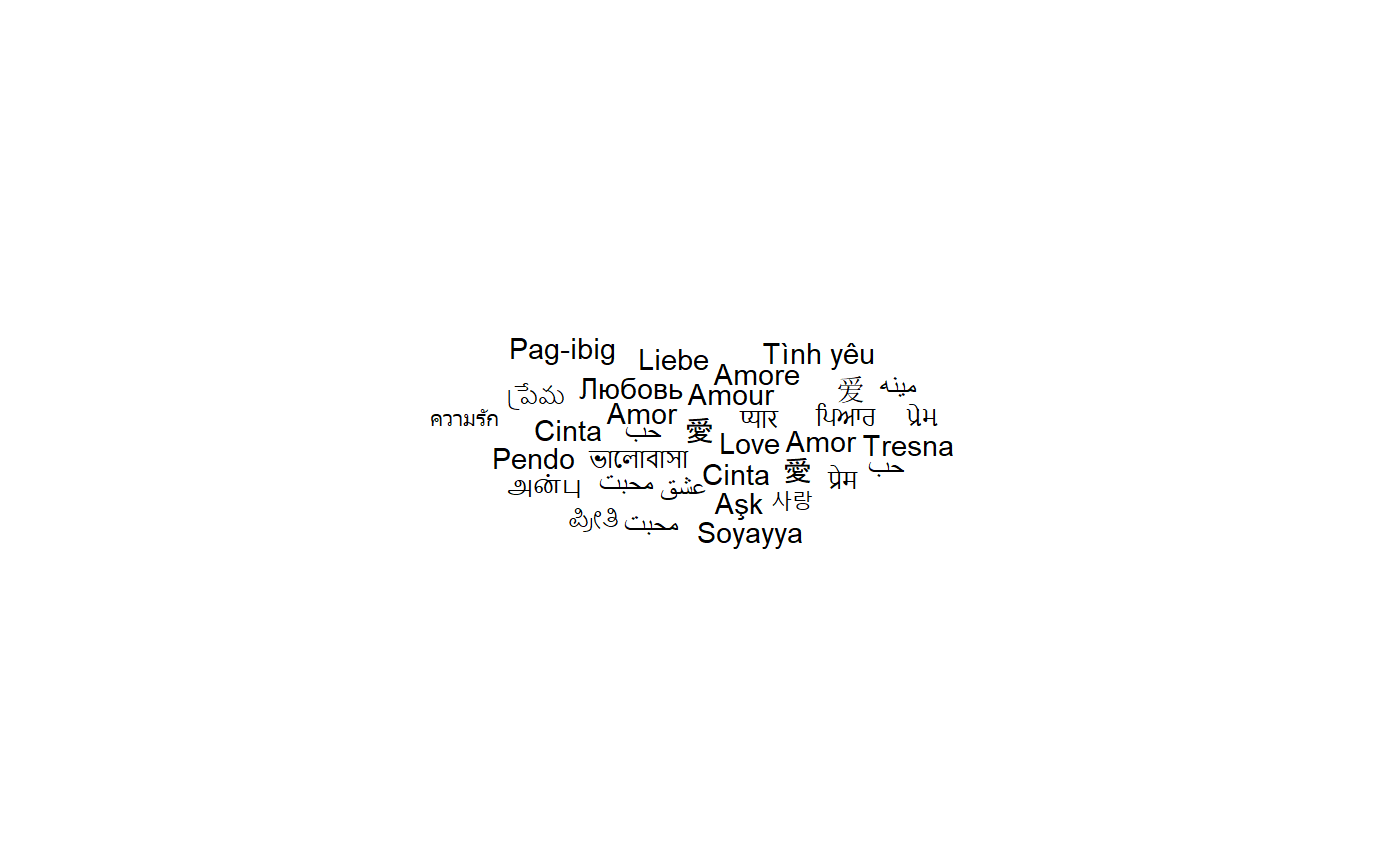# Word cloud and text size

So far all the words had the same size because we do not specify a size aesthetic. If we use the total number of speakers, we obtain:

``````set.seed(42)
ggplot(love_words_small, aes(label = word, size = speakers)) +
geom_text_wordcloud() +
theme_minimal()``````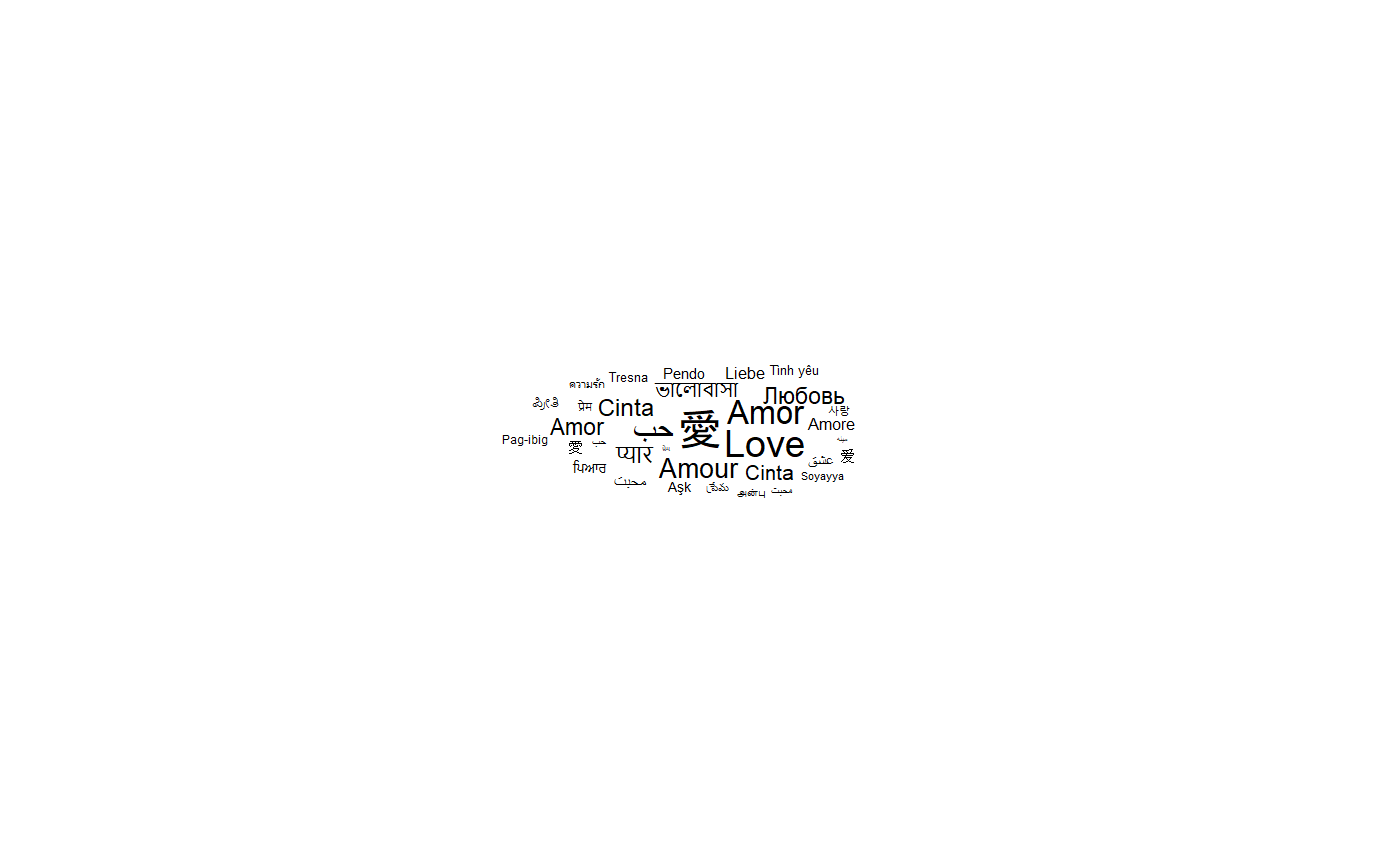The words are scaled according to the value of the size aesthetic, the number of speakers here. There are several classical choices for the scaling: the font size could be chosen proportional to the value or to the square root of the value so that the area of a given character is respectively proportional to the square of the value or the value itself. By default, `ggplot2` uses the square root scaling but does not map a value of \(0\) to \(0\).

In order to obtain a true proportionality (and a better font size control), one can use the `scale_size_area()` scale:

``````set.seed(42)
ggplot(love_words_small, aes(label = word, size = speakers)) +
geom_text_wordcloud() +
scale_size_area(max_size = 30) +
theme_minimal()``````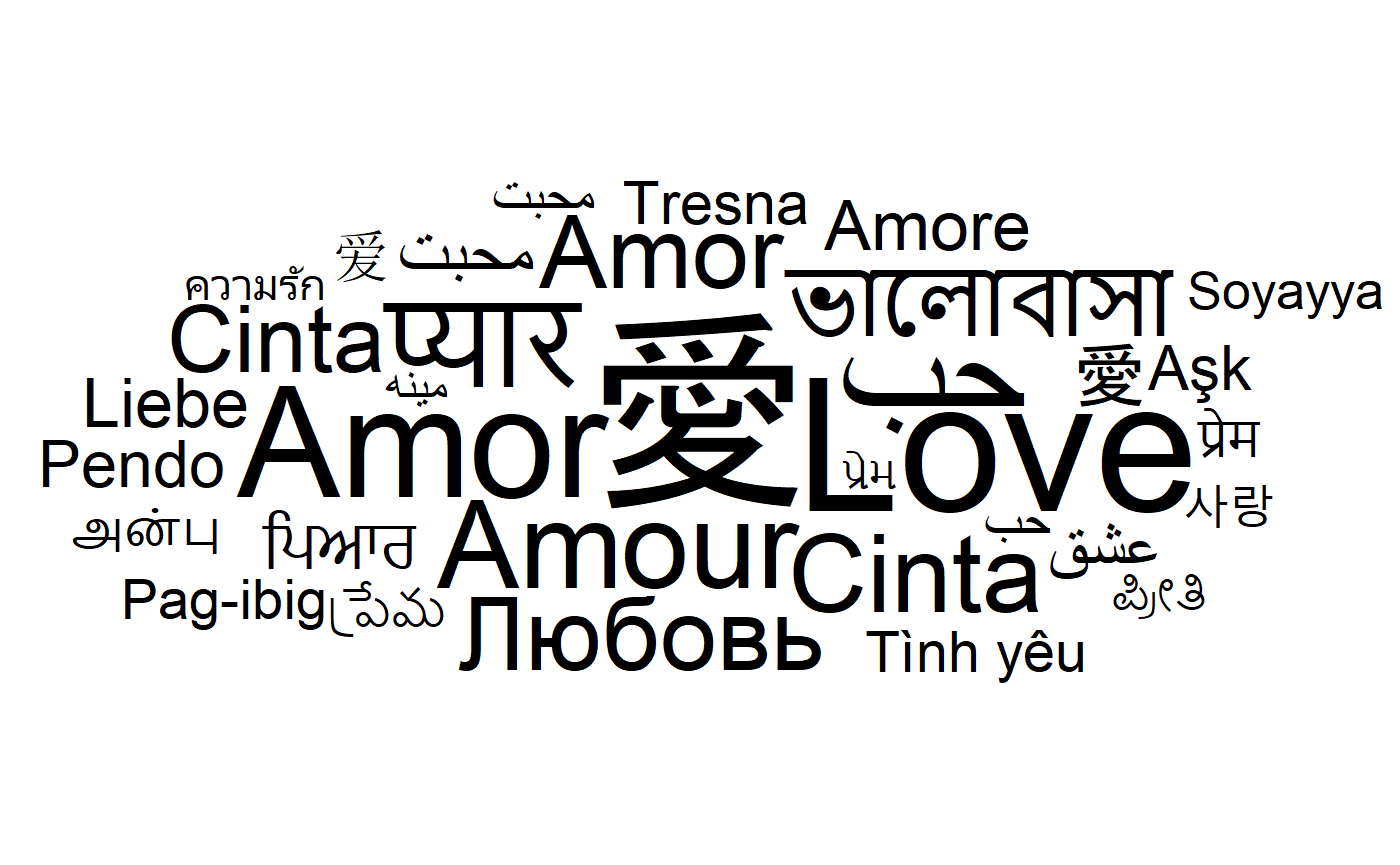It turns out that both `wordcloud` and `wordcloud2` default to a linear scaling between the value and the font size. This can be obtained with the `scale_radius()` scale:

``````set.seed(42)
ggplot(love_words_small, aes(label = word, size = speakers)) +
geom_text_wordcloud() +
scale_radius(range = c(0, 30), limits = c(0, NA)) +
theme_minimal()``````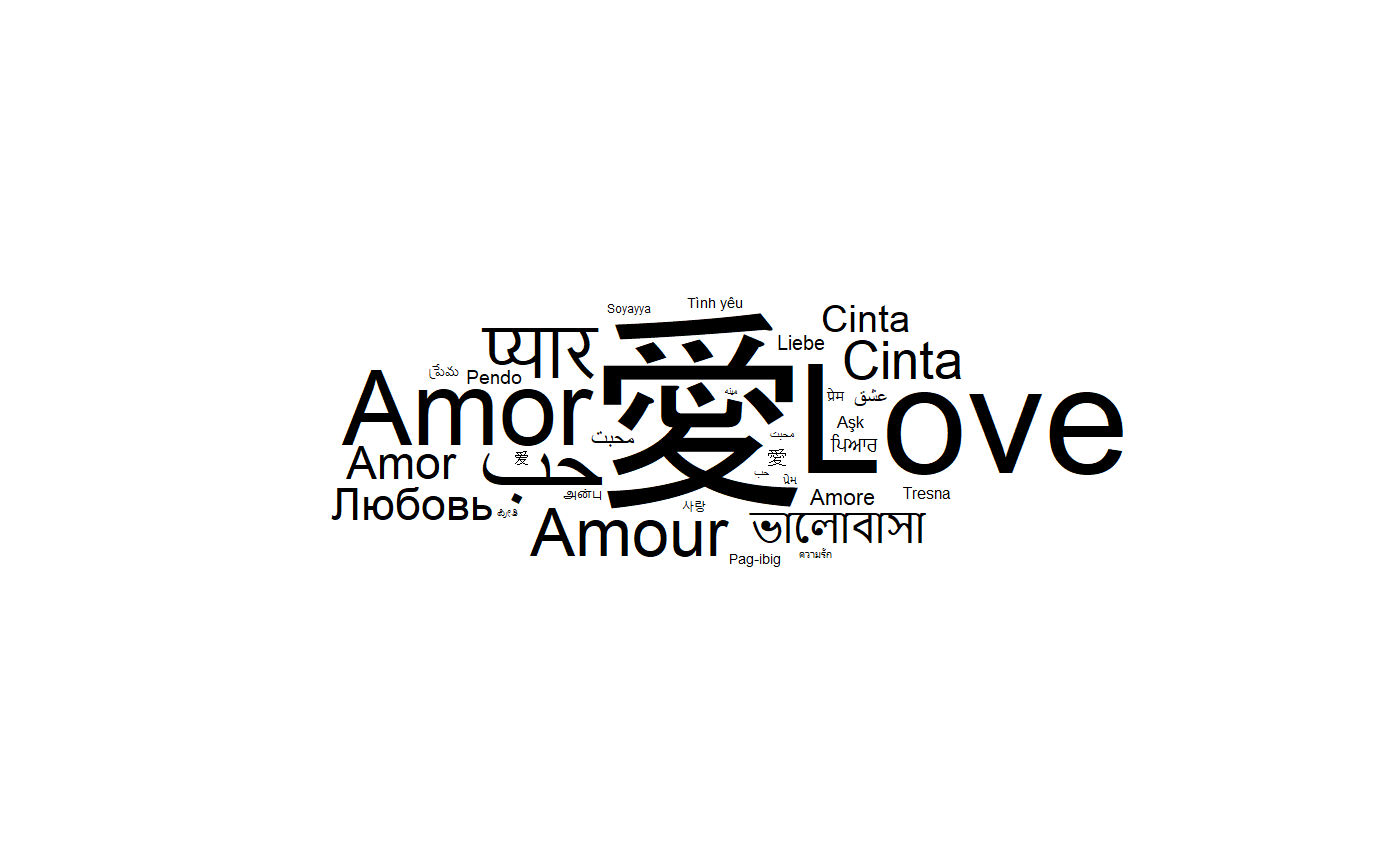# Word cloud and text area

As explained before, by default, this is the size of the font which is proportional to the square root of the value of the size aesthetic. This is a natural choice for a shape as the area of the shape will be proportional to the raw size aesthetic but not necessarily for texts with different lengths. In `ggwordcloud2`, there is an option, `area_corr` to scale the font of each label so that the text area is a function of the raw size aesthetic when used in combination with `scale_size_area`:

``````set.seed(42)
ggplot(love_words_small, aes(label = word, size = speakers)) +
geom_text_wordcloud(area_corr = TRUE) +
scale_size_area(max_size = 50) +
theme_minimal()``````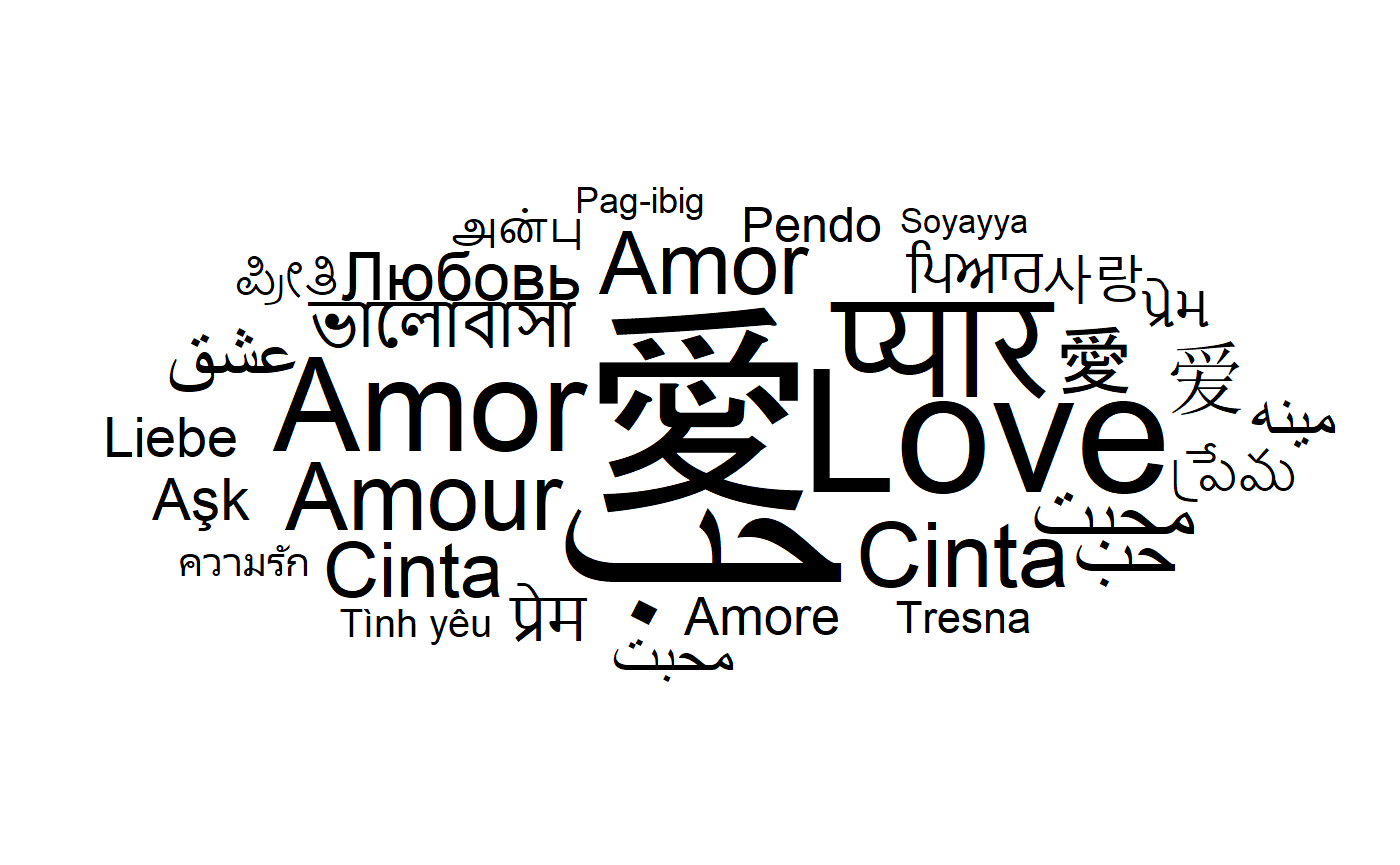One can equivalently use the `geom_text_wordcloud_area` geom:

``````set.seed(42)
ggplot(love_words_small, aes(label = word, size = speakers)) +
geom_text_wordcloud_area() +
scale_size_area(max_size = 50) +
theme_minimal()``````By default, the area is proportional to the raw size aesthetic. To better match the human area perception, one can use the `power_trans` scale with a factor of \(1/.7\):

``````set.seed(42)
ggplot(love_words_small, aes(label = word, size = speakers)) +
geom_text_wordcloud_area() +
scale_size_area(max_size = 50, trans = power_trans(1/.7)) +
theme_minimal()``````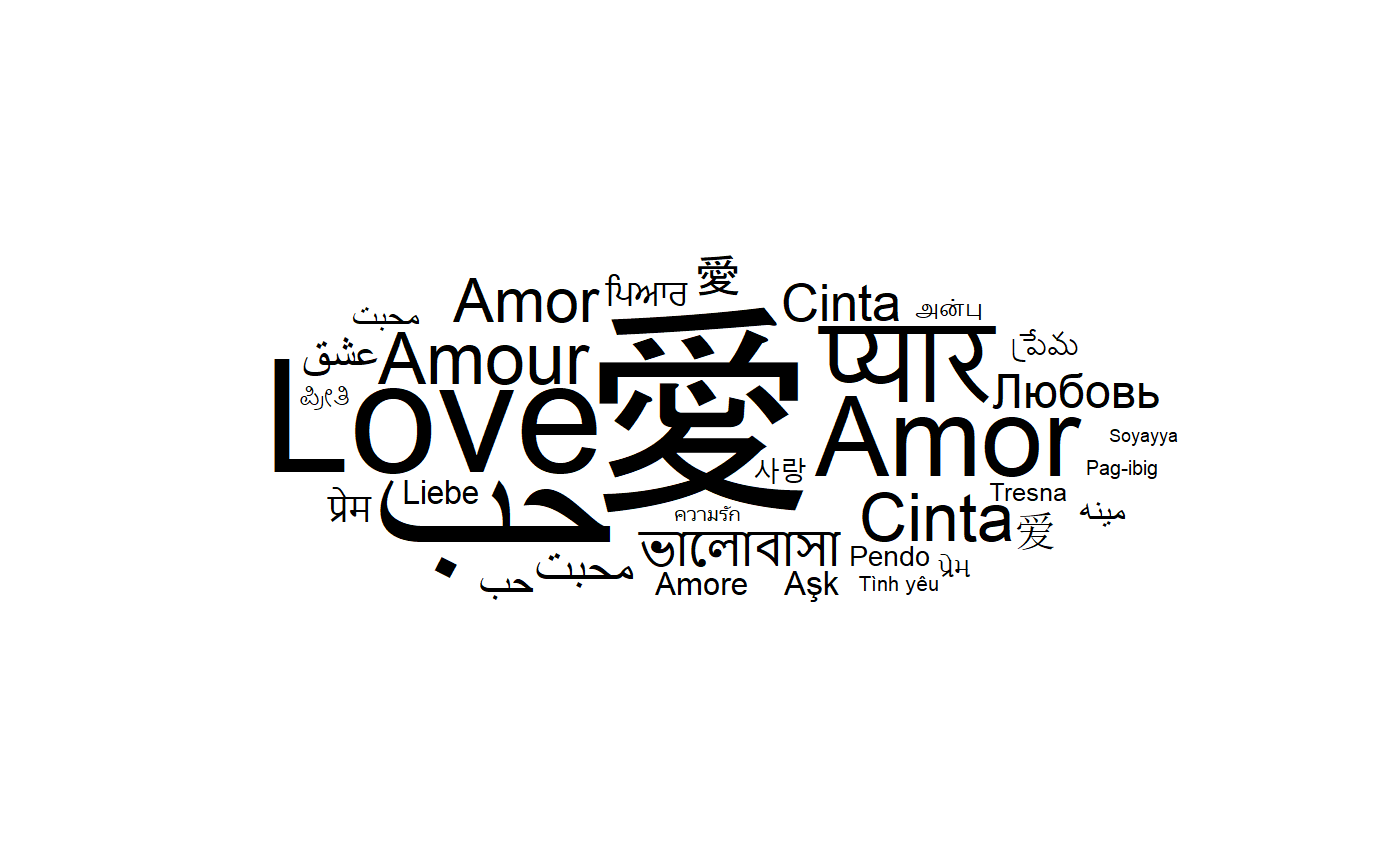# Word cloud with too many words

The non overlapping algorithm may fail to place some words due to a lack of space. By default, those words are displayed at the center of the word cloud and comes with a warning.

``````set.seed(42)
ggplot(love_words_small, aes(label = word, size = speakers)) +
geom_text_wordcloud_area() +
scale_size_area(max_size = 80) +
theme_minimal()
#> Warning in wordcloud_boxes(data_points = points_valid_first, boxes = boxes, :
#> Some words could not fit on page. They have been placed at their original
#> positions.``````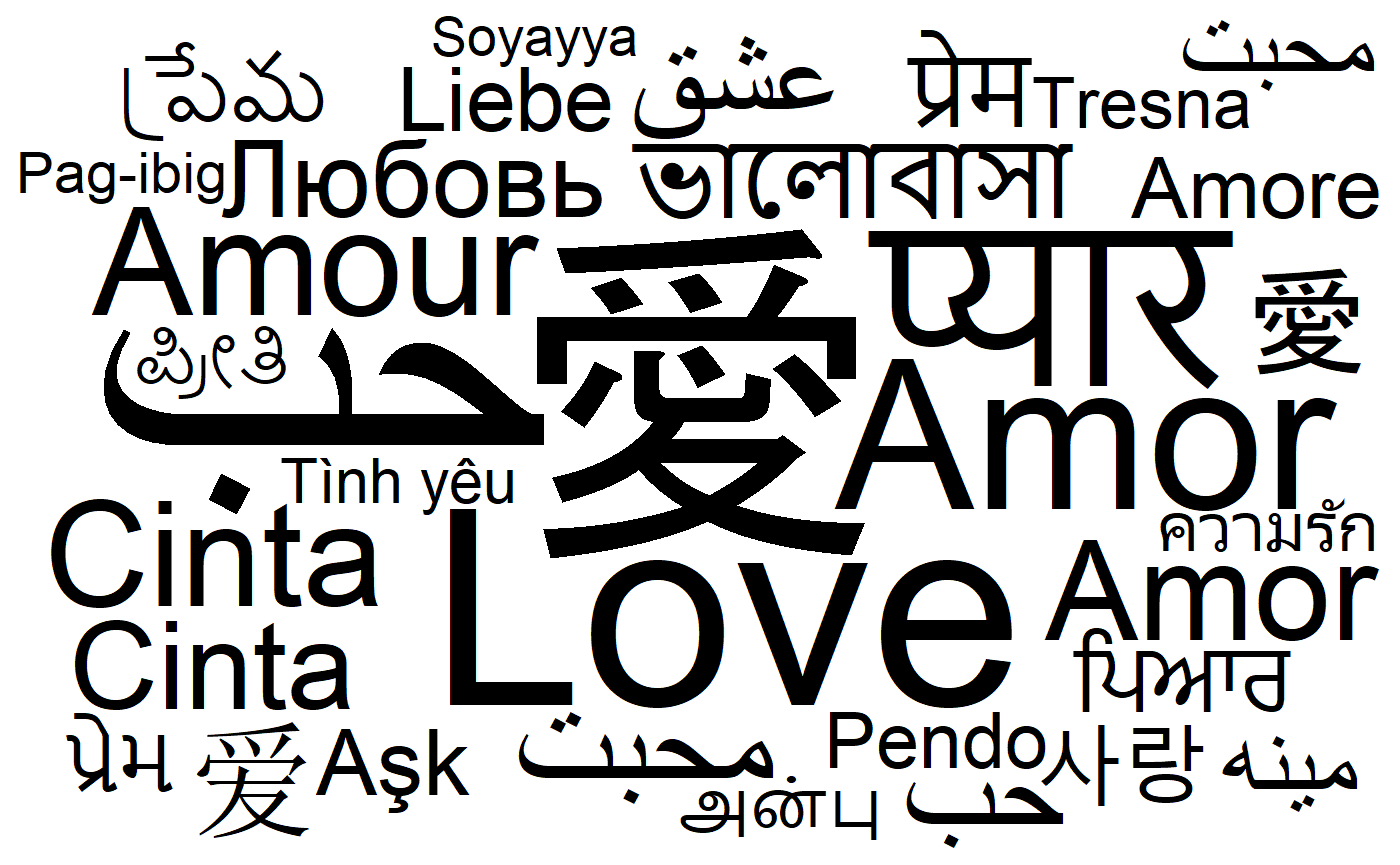It is up to the user to avoid this issue by either removing some words or changing the size scale. One can also chose to remove those words using the `rm_outside` option:

``````set.seed(42)
ggplot(love_words_small, aes(label = word, size = speakers)) +
geom_text_wordcloud_area(rm_outside = TRUE) +
scale_size_area(max_size = 80) +
theme_minimal()
#> Warning in wordcloud_boxes(data_points = points_valid_first, boxes = boxes, :
#> Some words could not fit on page. They have been removed.``````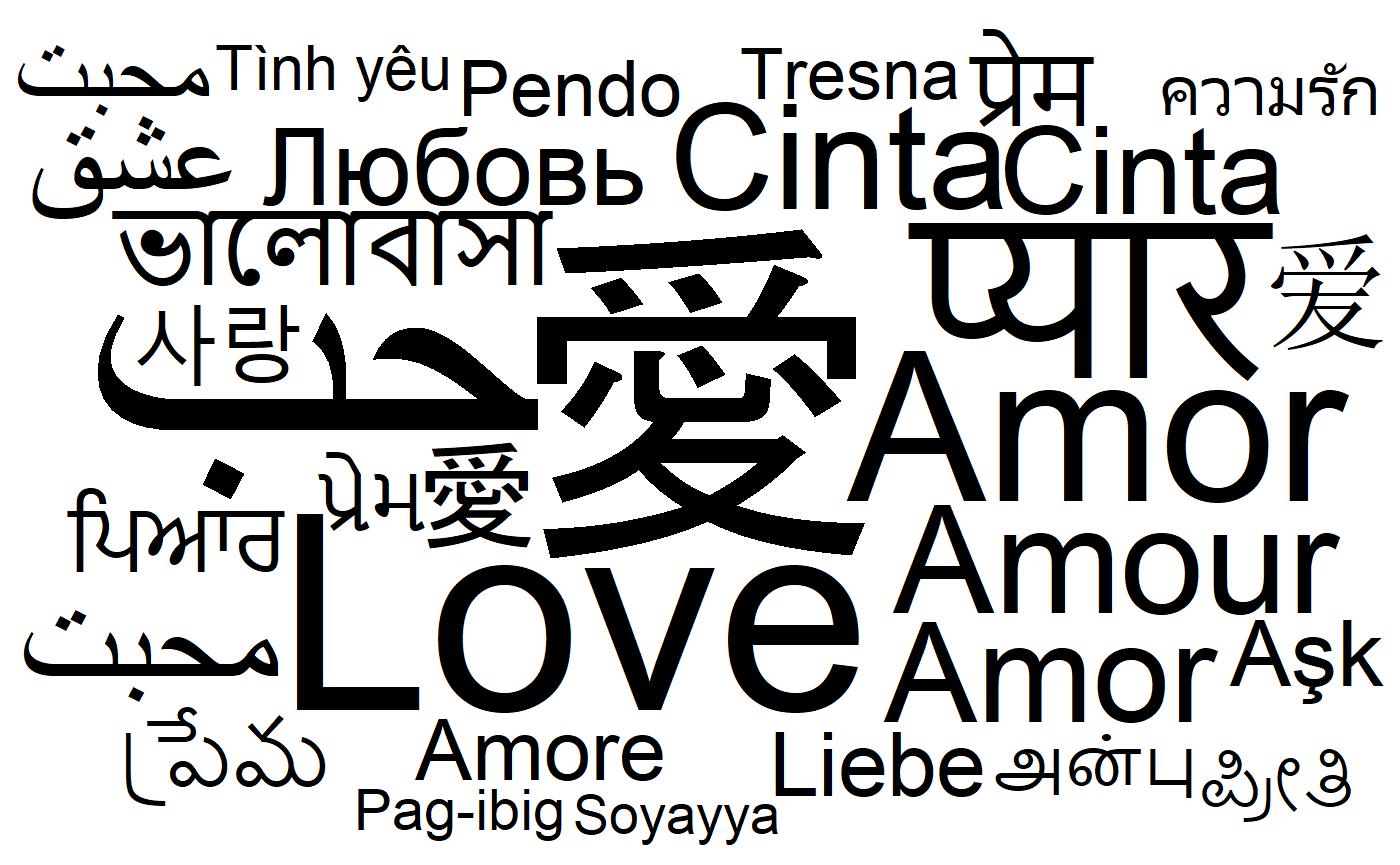# Word cloud and rotation

The words can be rotated by setting the `angle` aesthetic. For instance, one can use a rotation of 90 degrees for a random subset of 40 % of the words:

``````library(dplyr, quietly = TRUE)
#>
#> Attachement du package : 'dplyr'
#> Les objets suivants sont masqués depuis 'package:stats':
#>
#>     filter, lag
#> Les objets suivants sont masqués depuis 'package:base':
#>
#>     intersect, setdiff, setequal, union
love_words_small <- love_words_small %>%
mutate(angle = 90 * sample(c(0, 1), n(), replace = TRUE, prob = c(60, 40)))``````
``````set.seed(42)
ggplot(love_words_small, aes(
label = word, size = speakers,
angle = angle
)) +
geom_text_wordcloud_area() +
scale_size_area(max_size = 40) +
theme_minimal()``````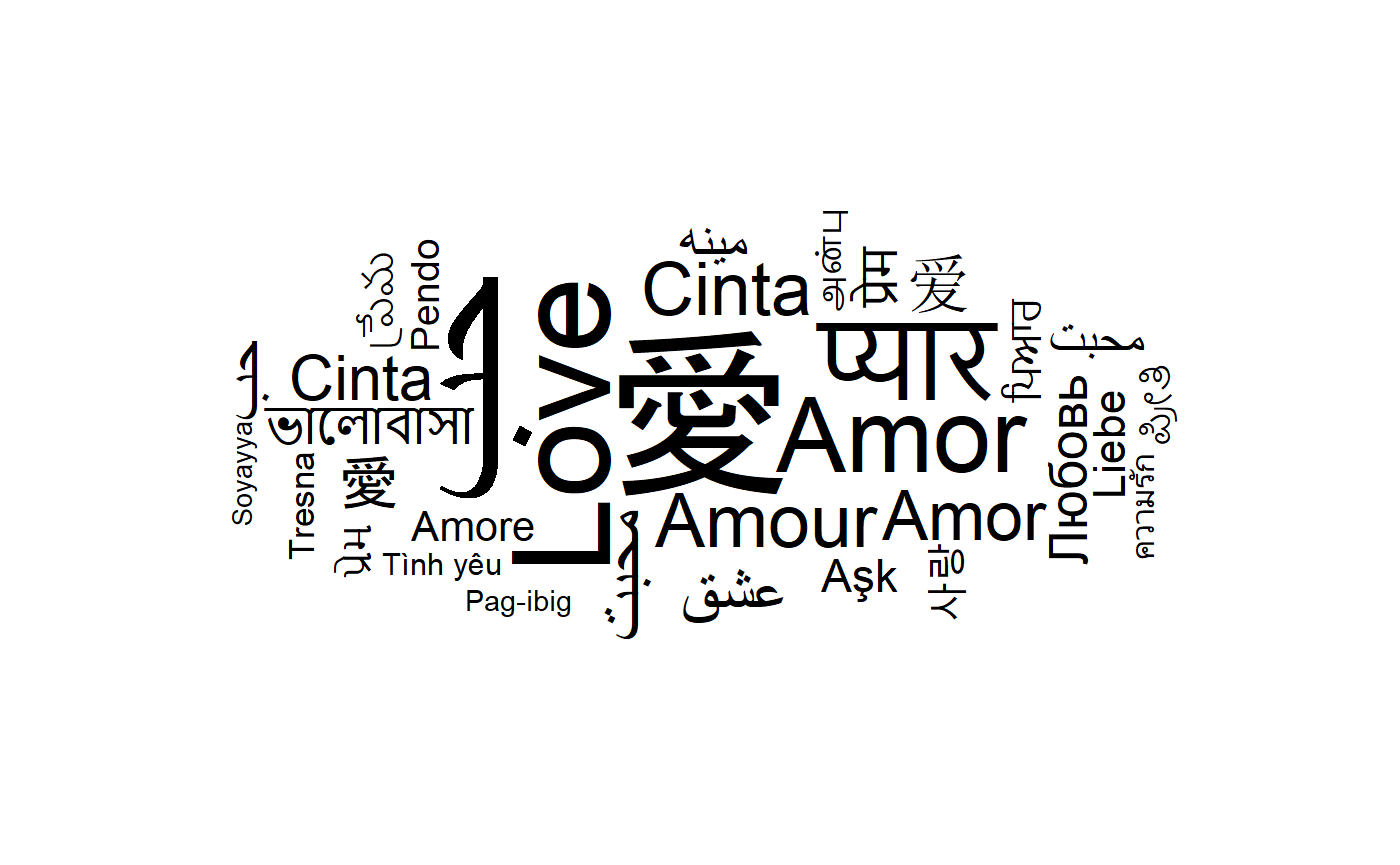`ggwordcloud` is not restricted to rotation of 90 degrees:

``````love_words_small <- love_words_small %>%
mutate(angle = 45 * sample(-2:2, n(), replace = TRUE, prob = c(1, 1, 4, 1, 1)))``````
``````set.seed(42)
ggplot(love_words_small, aes(
label = word, size = speakers,
angle = angle
)) +
geom_text_wordcloud_area() +
scale_size_area(max_size = 40) +
theme_minimal()``````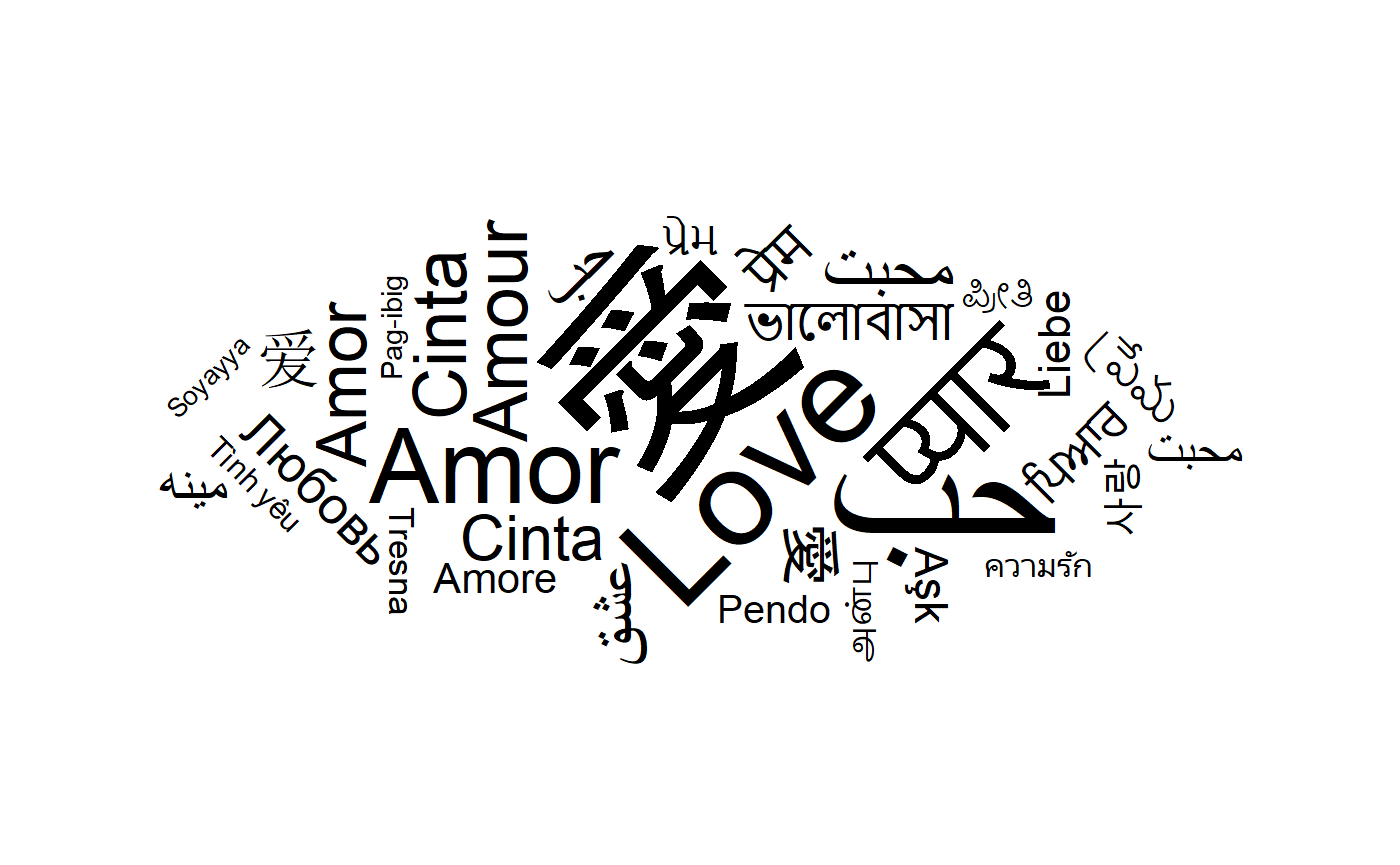# Word cloud and eccentricity

The `ggwordcloud` algorithm moves the text around a spiral until it finds a free space for it. This spiral has by default a vertical eccentricity of .65, so that the spiral is 1/.65 wider than taller.

``````set.seed(42)
ggplot(love_words_small, aes(label = word, size = speakers)) +
geom_text_wordcloud_area() +
scale_size_area(max_size = 40) +
theme_minimal()``````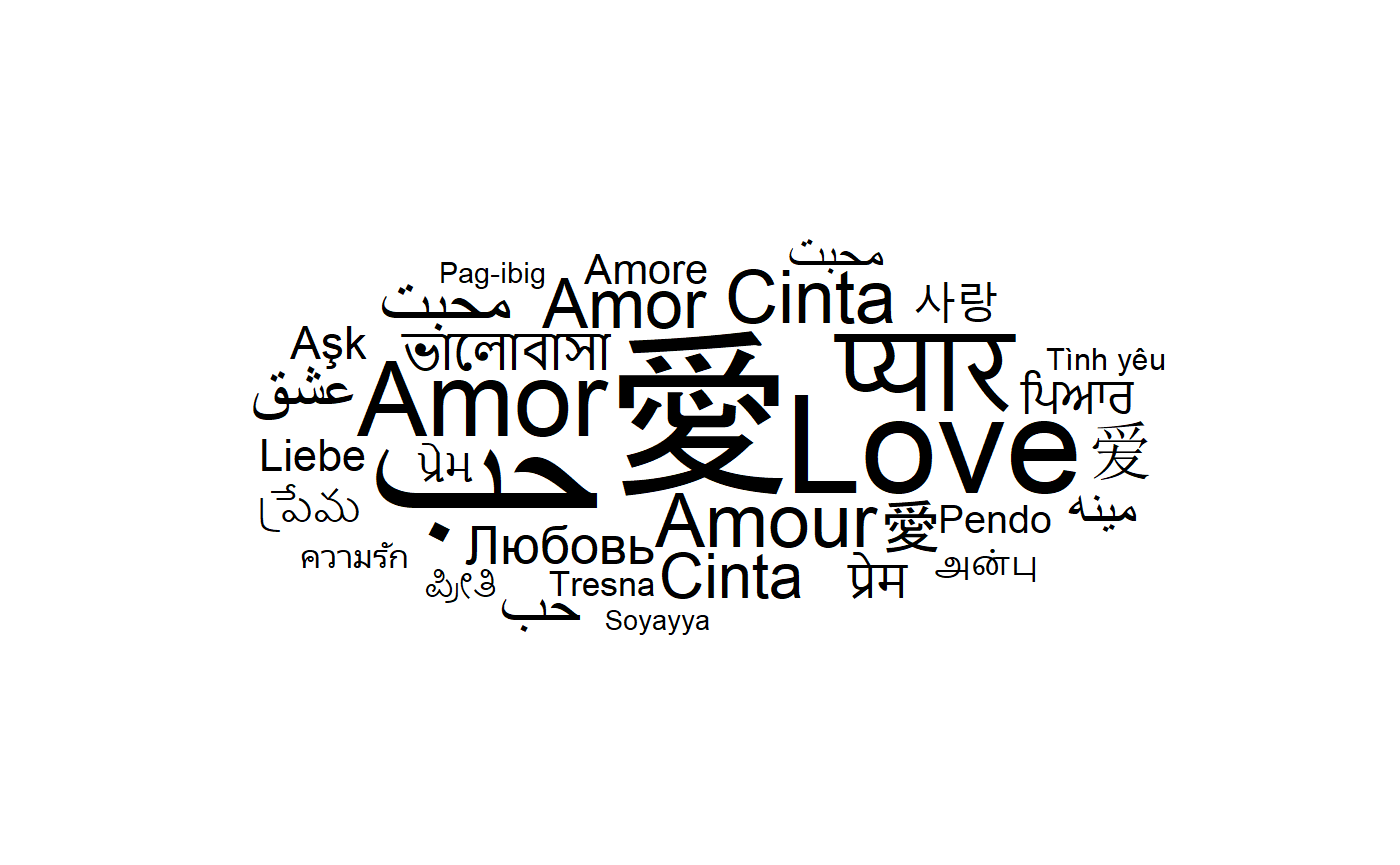This can be changed using the `eccentricity` parameter:

``````set.seed(42)
ggplot(love_words_small, aes(label = word, size = speakers)) +
geom_text_wordcloud_area(eccentricity = 1) +
scale_size_area(max_size = 40) +
theme_minimal()``````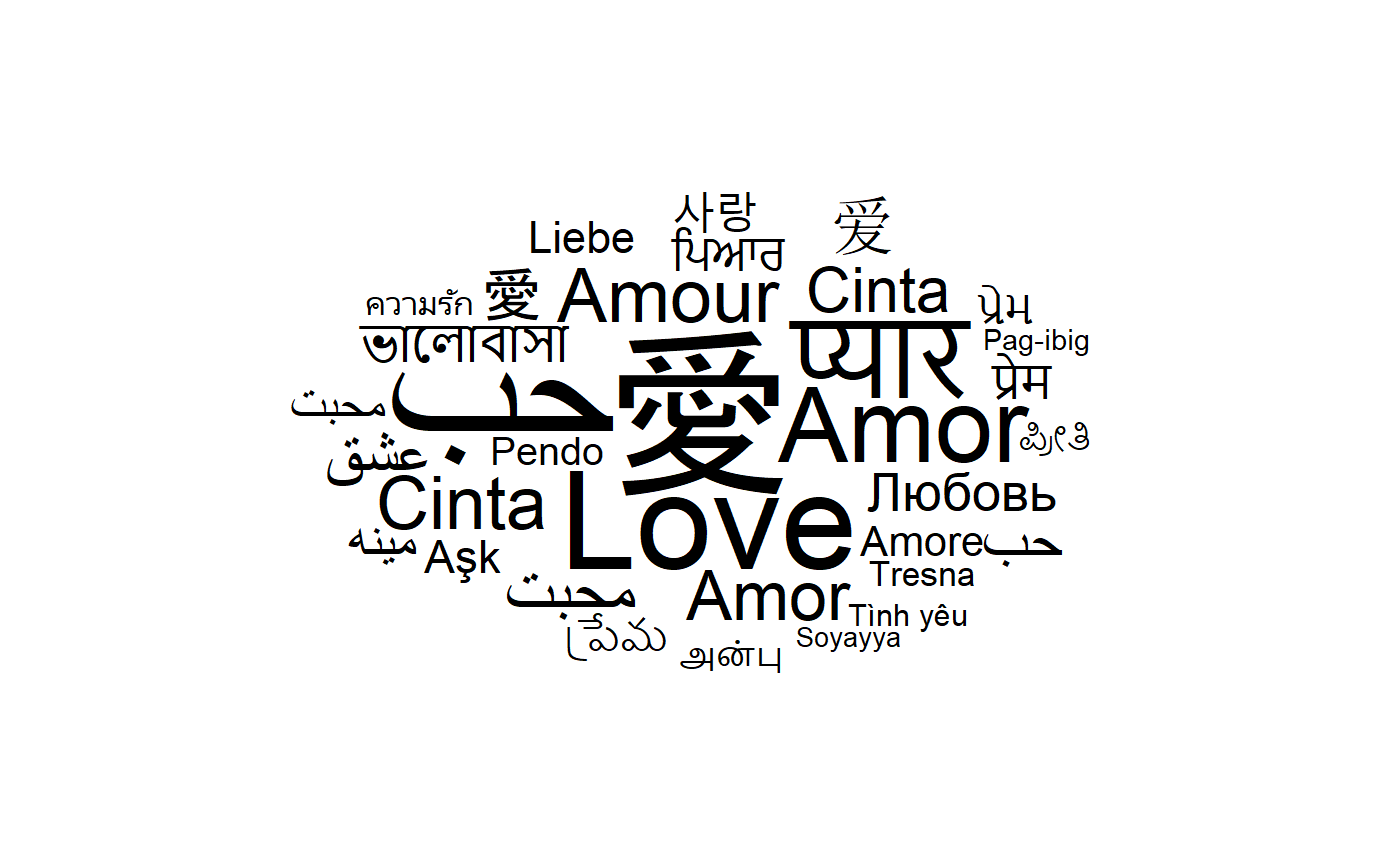``````set.seed(42)
ggplot(love_words_small, aes(label = word, size = speakers)) +
geom_text_wordcloud_area(eccentricity = .35) +
scale_size_area(max_size = 40) +
theme_minimal()``````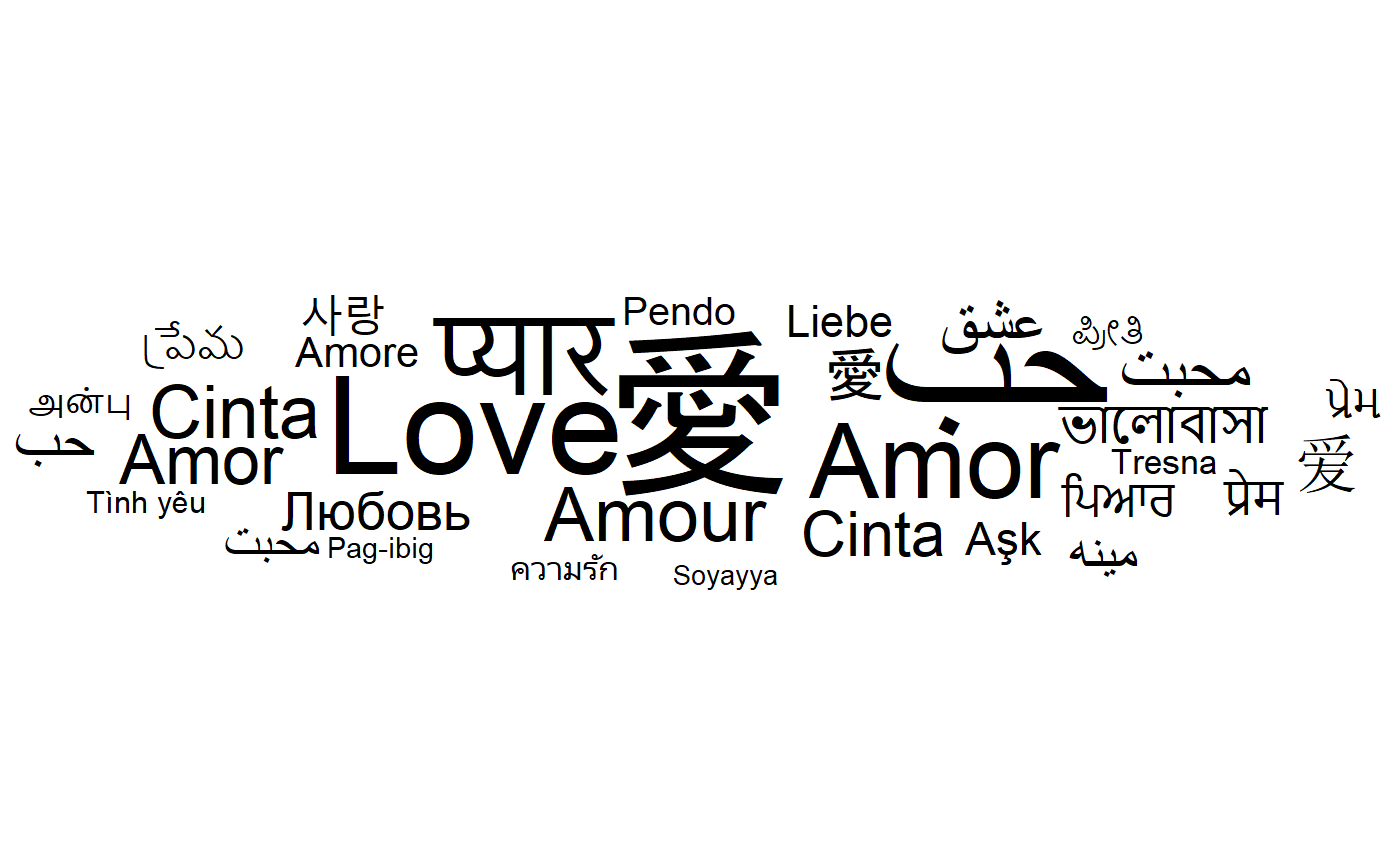# Word cloud and shape

The base shape of `ggwordcloud` is a circle: the words are place by following a circle spiral. This base shape `circle` can be change to others (`cardioid`, `diamond`, `square`, `triangle-forward`, `triangle-upright`, `pentagon` or `star`) using the `shape` option.

``````for (shape in c(
"circle", "cardioid", "diamond",
"square", "triangle-forward", "triangle-upright",
"pentagon", "star"
)) {
set.seed(42)
print(ggplot(love_words_small, aes(label = word, size = speakers)) +
geom_text_wordcloud_area(shape = shape) +
scale_size_area(max_size = 40) +
theme_minimal() + ggtitle(shape))
}``````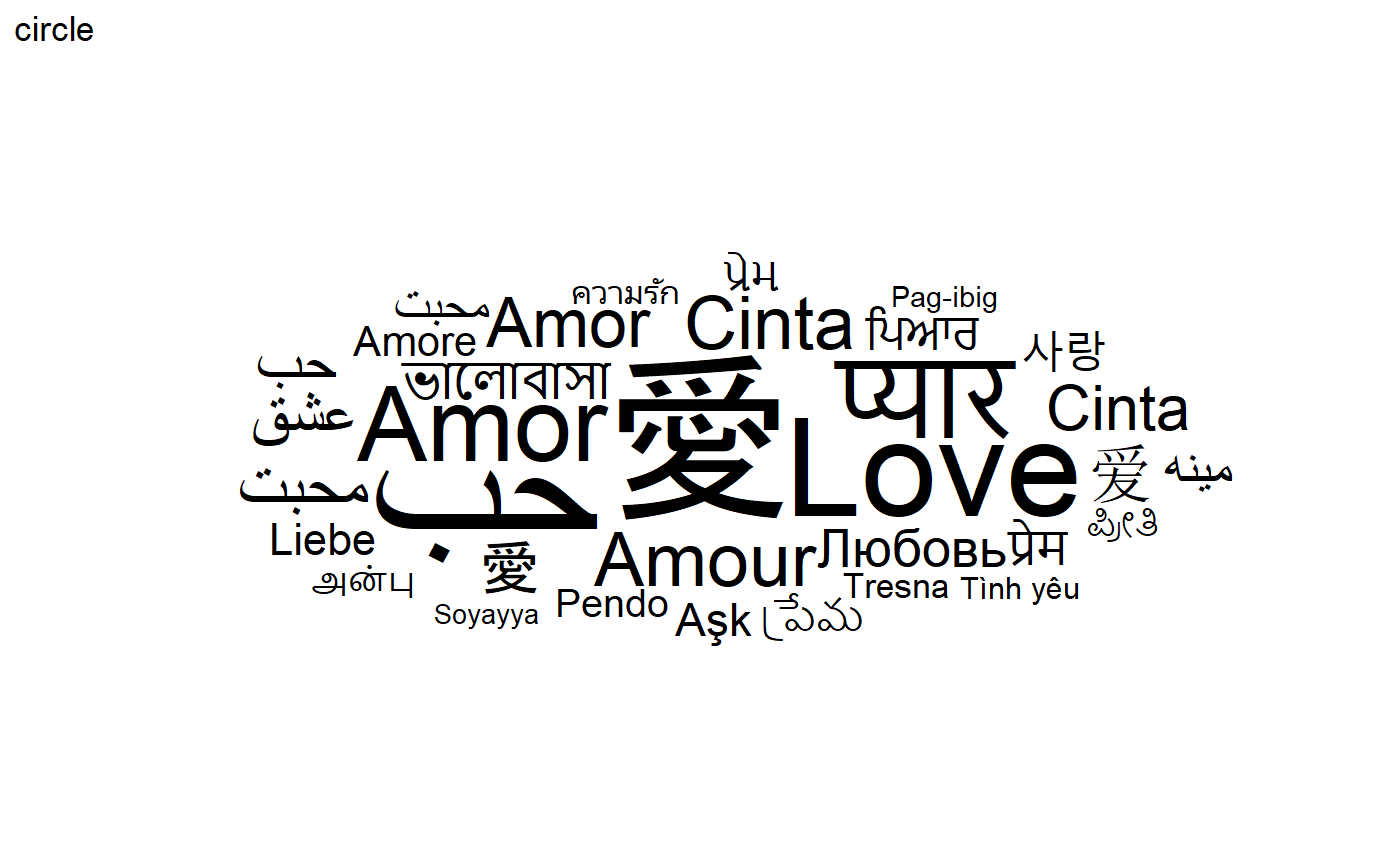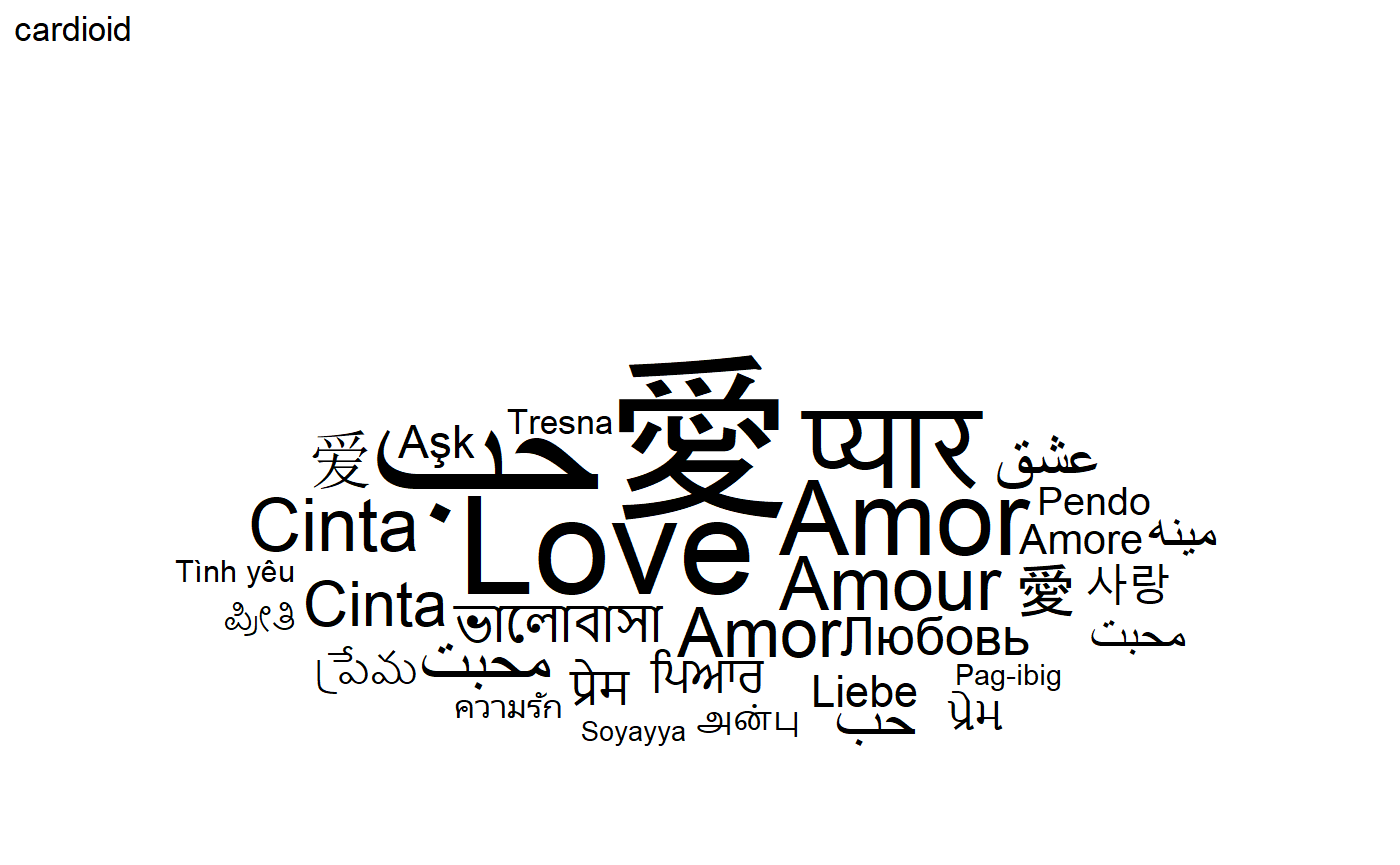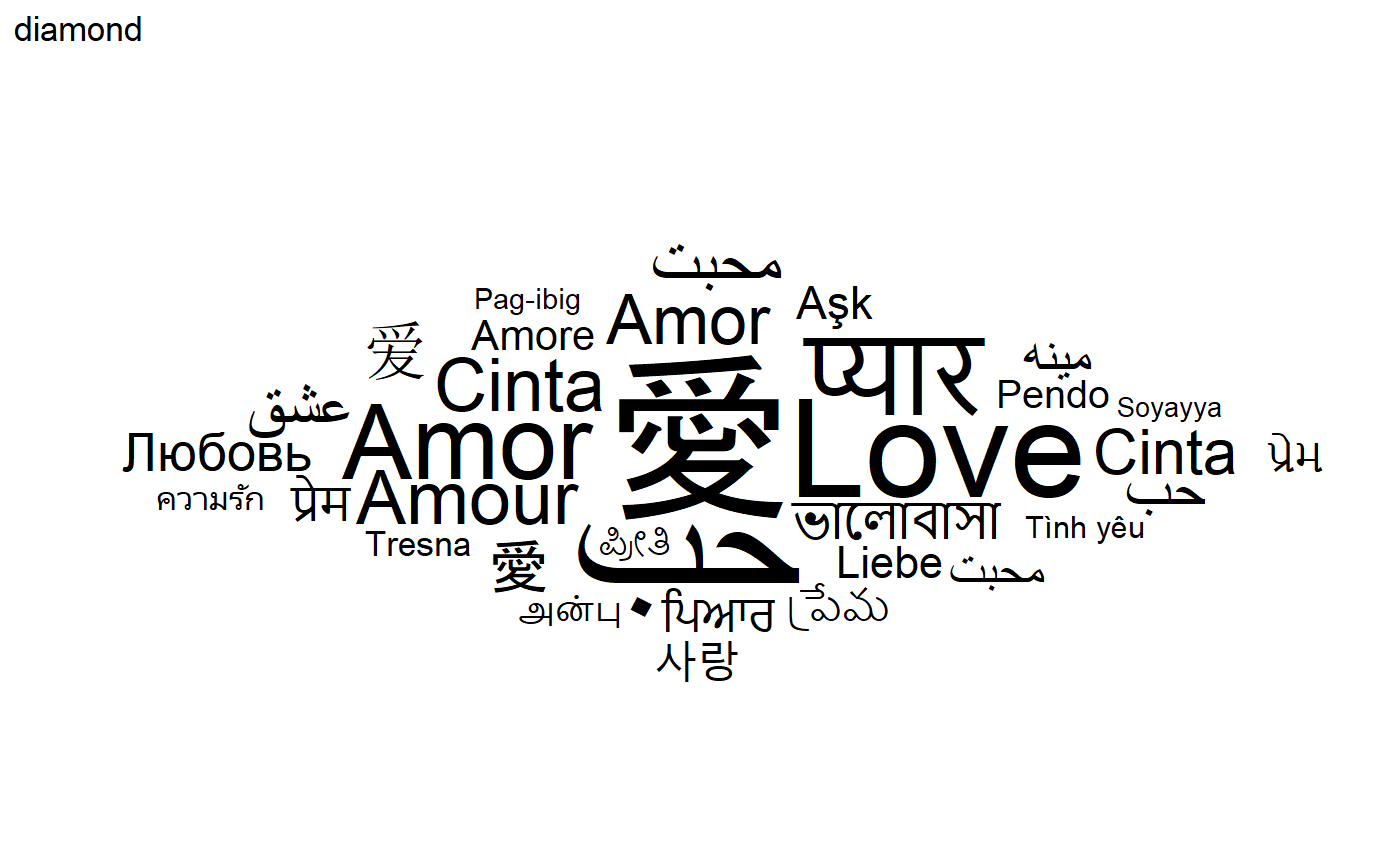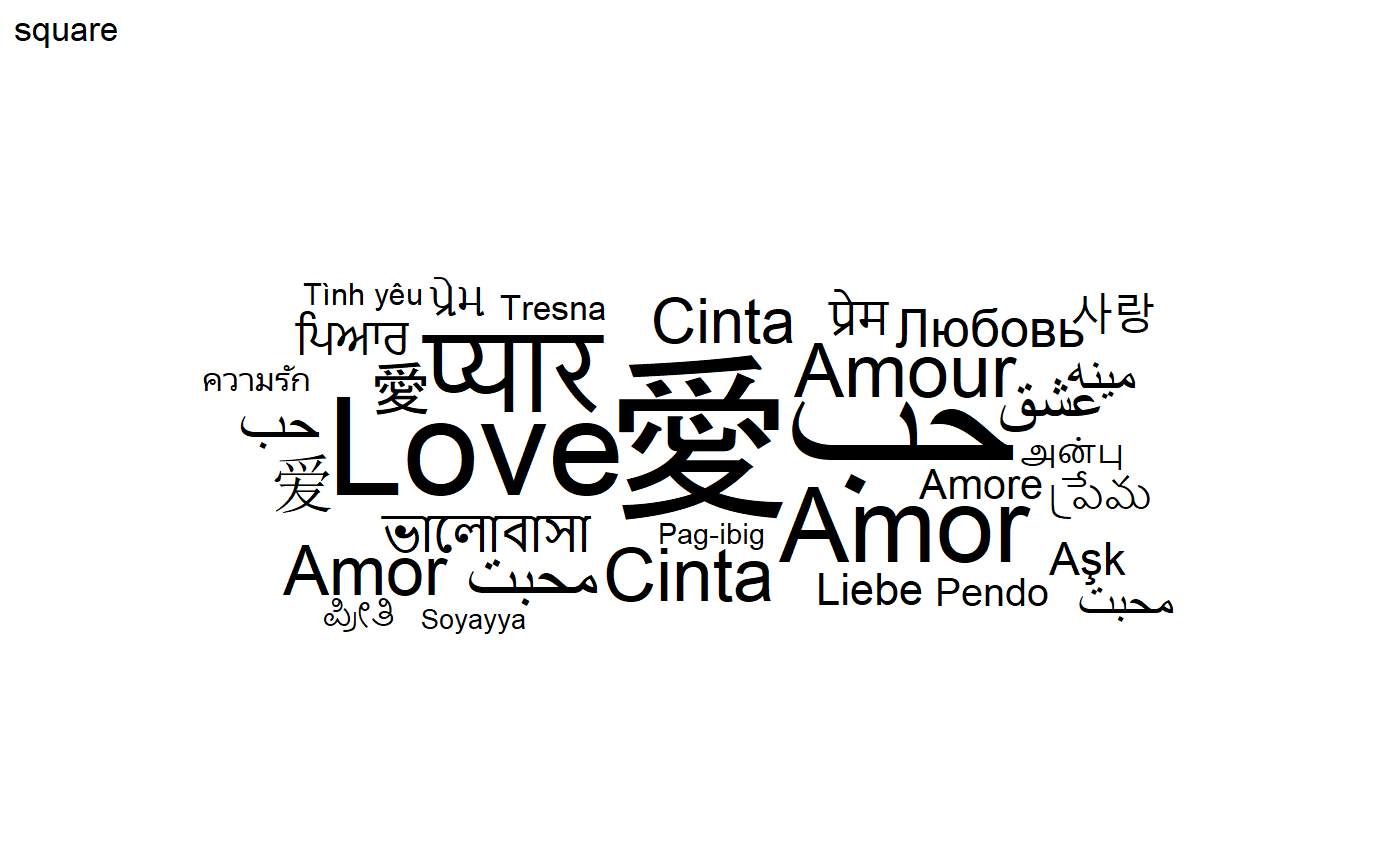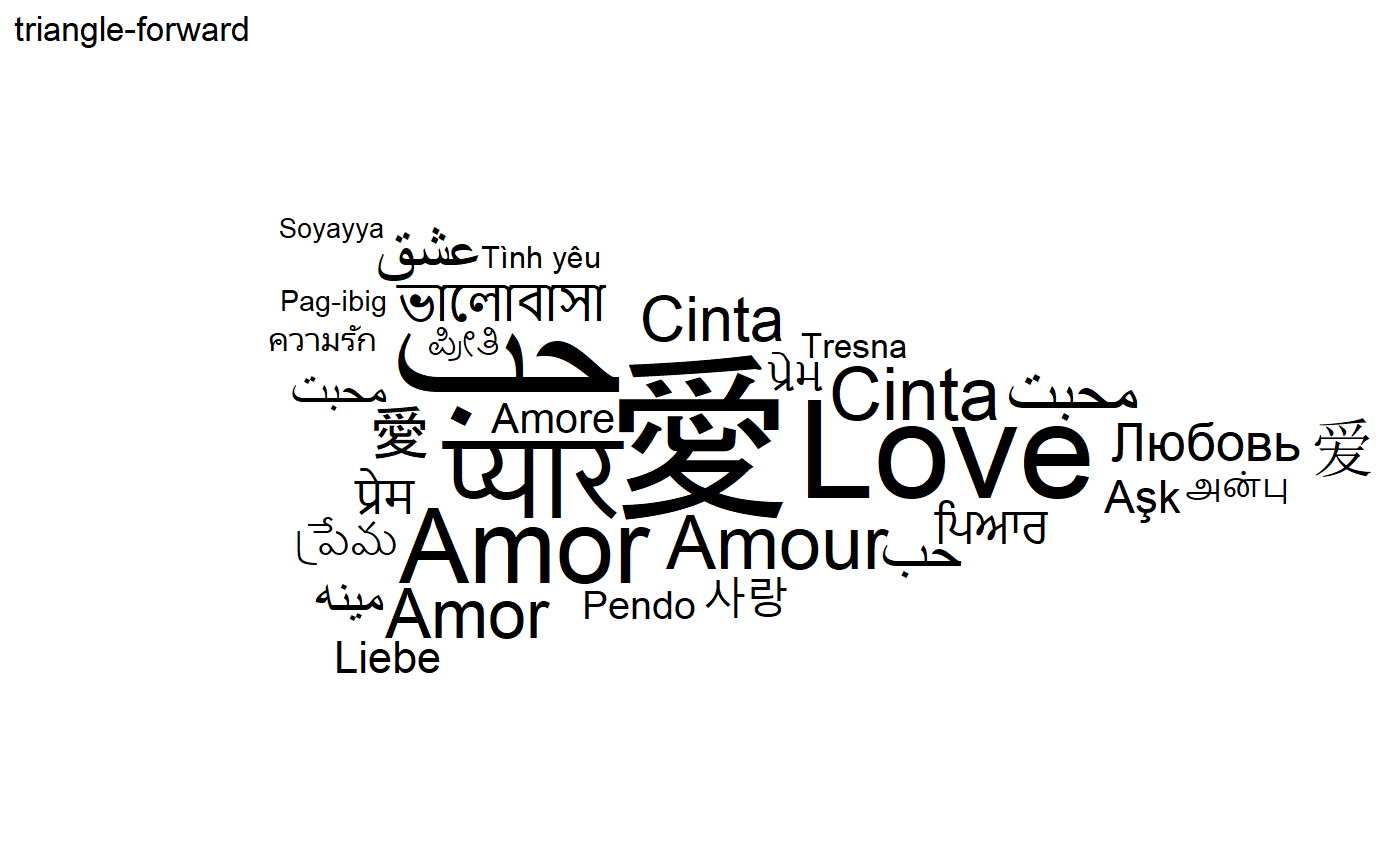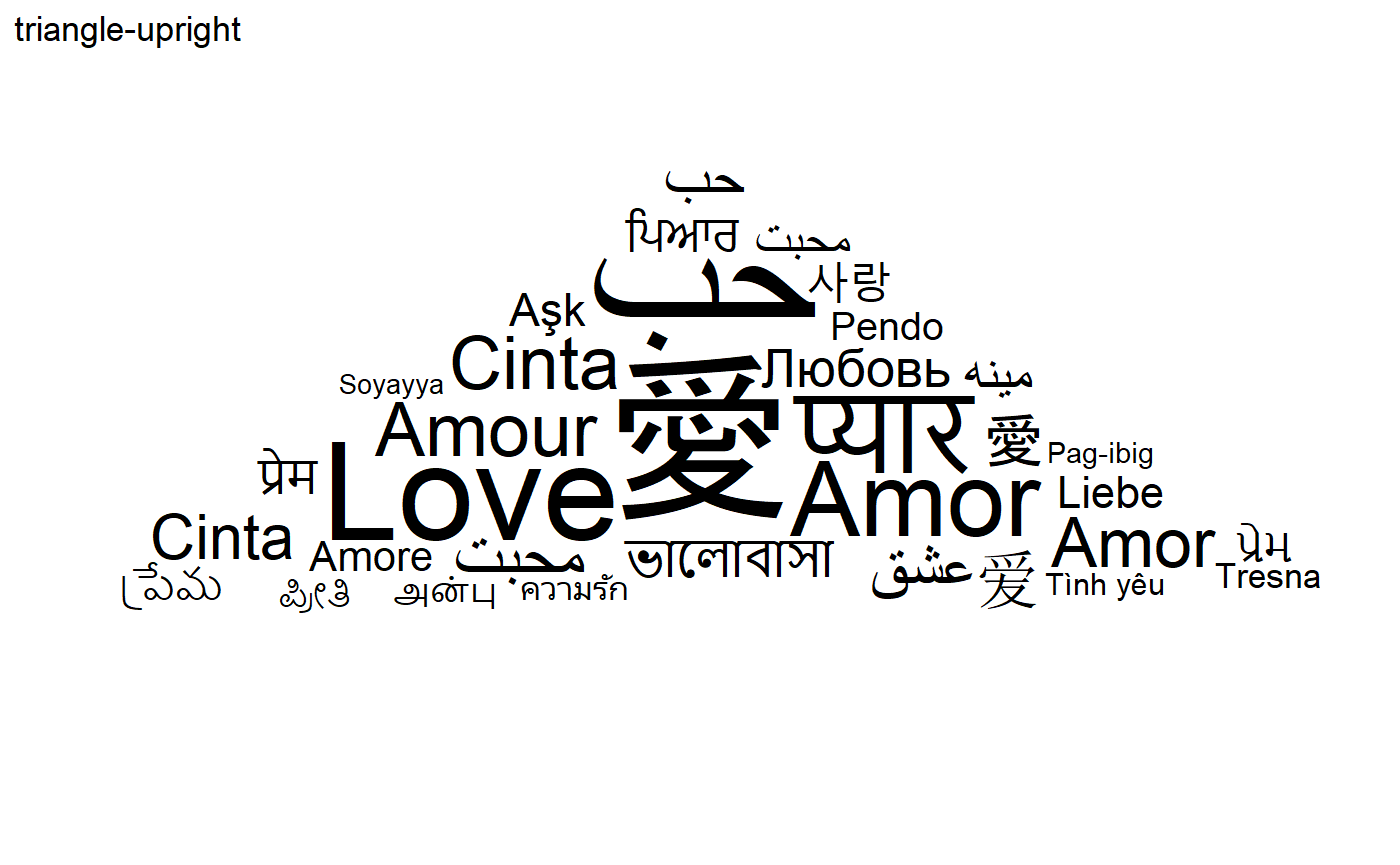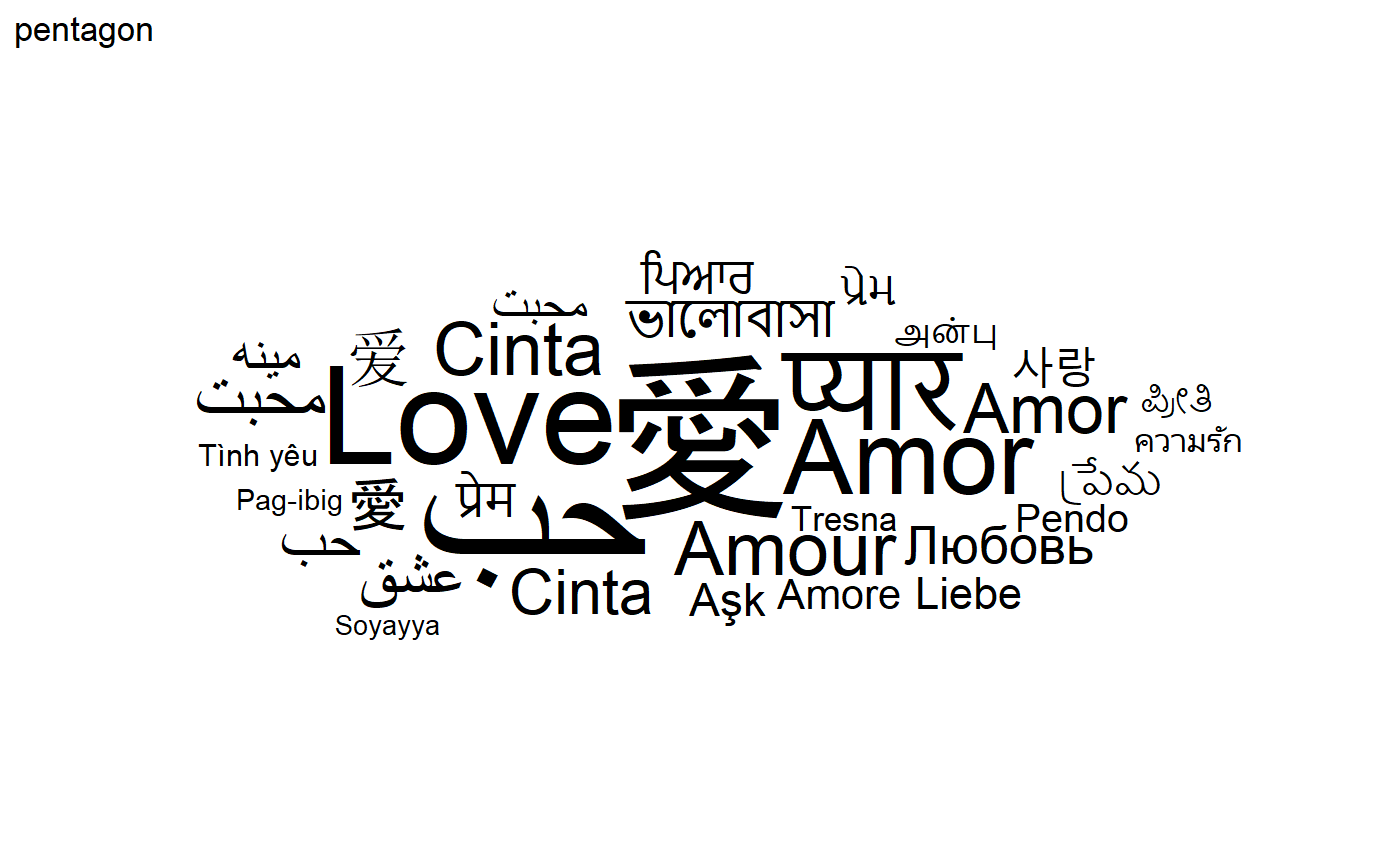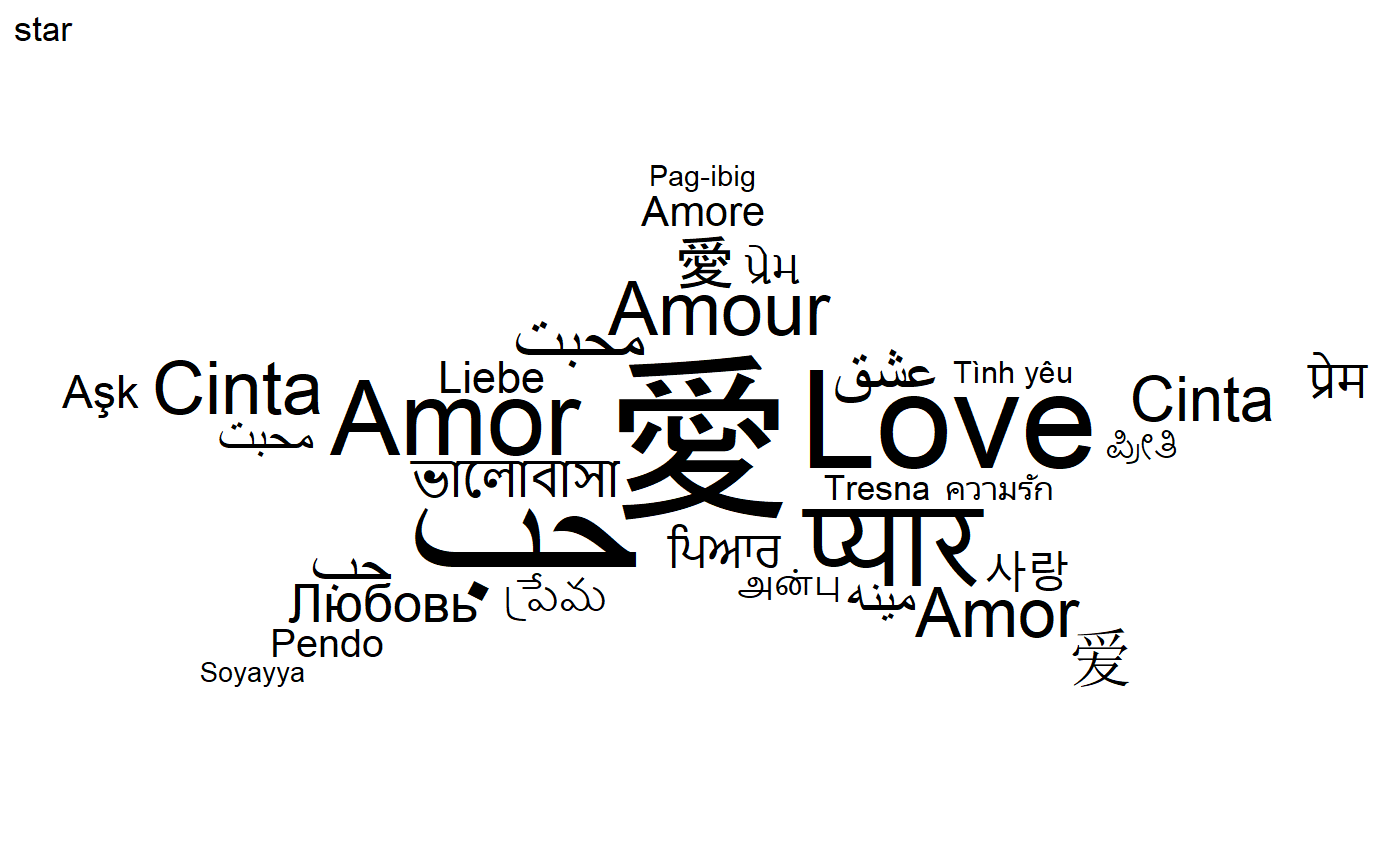# Word cloud and color

A color can be assign to each word using the color aesthetic. For instance, one can assign a random factor to each word:

``````set.seed(42)
ggplot(
love_words_small,
aes(
label = word, size = speakers,
color = factor(sample.int(10, nrow(love_words_small), replace = TRUE)),
angle = angle
)
) +
geom_text_wordcloud_area() +
scale_size_area(max_size = 40) +
theme_minimal()``````